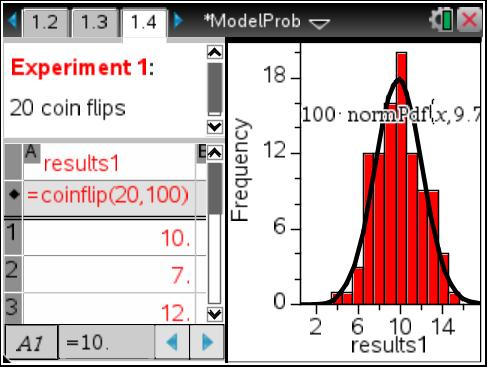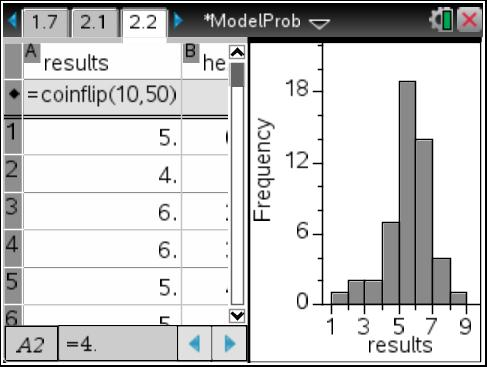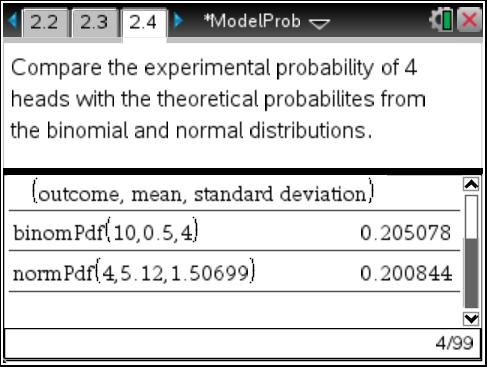# Activities

••• ##### Subject Area

• Math: Algebra II: Data Analysis

• ##### Author9-12

60 Minutes

• ##### Device
• TI-Nspire™ Navigator™
• TI-Nspire™
• TI-Nspire™ CAS
•TI-Nspire™ CX CAS/CX II CAS
•TI-Nspire™ CX/CX II
• ##### Software

TI-Nspire™
TI-Nspire™ CAS

3.2

## Modeling Probabilities#### Activity Overview

This activity approaches probability distributions as functions taking information about a dataset as inputs and outputting a set of probabilities. This is analogous to the familiar process of choosing a function to model a set of x-y pairs. Students use simulations to explore increasing number of trials. The normal distribution is introduced, compared with the binomial distribution, and applied.

#### Key Steps

•In Problem 1, students simulate a binomial experiment using the custom function coinflip. When the simulation runs, each row of the table displays the number of heads that occurred for a trial. Students repeat the process using different numbers of trials to determine the shape of the histogram.

•In Problem 2, students explore probability distributions. They simulate 10 flips, for 50 trials in Column A. Then they use a histogram to determine and record the number of times an outcome occurs in the experiment in Column C. Lastly, students will use a formula to calculate the experimental probabilities.

•Students will compare the experimental probabilities with the probabilities calculated with the binomPdf and normPdf commands to determine which distribution best models each situation.